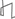Research Abstracts Home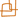CSAIL Digital Archive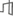Research Activities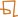CSAIL HomeResearch Abstracts - 2007IntroductionPerception & LearningPhysical, Biological & Social SystemsSystemsTheory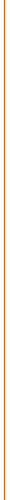### PTASs for Planar Graphs and Generalizations

#### Erik D. Demaine & MohammadTaghi Hajiaghayi

##### Context

There are two main general approaches for designing polynomial-time approximation schemes (PTASs) for problems on planar graphs and their generalizations. (A graph is planar if it can be drawn in the plane (or the sphere) without crossings. For definitions of other related graph classes, see the research abstract on Bidimensionality.)

Lipton and Tarjan [LT79,LT80] introduced the first approach, which is based on planar separators. The first step in this approach is to find a separator of O(√n) vertices or edges, where n is the size of the graph, whose removal splits the graph into two or more pieces each of which is a constant fraction smaller than the original graph. Then we recurse in each piece, building a recursion tree of separators, and stop when the pieces have some constant size such as 1/ε. The problem can be solved on these pieces by brute force, and then it remains to combine the solutions up the recursion tree. The induced error can often be bounded in terms of the total size of all separators, which in turn can be bounded by ε n. If the optimal solution is at least some constant factor times n, this approach often leads to a PTAS.

There are two limitations to this planar-separator approach. First, it requires that the optimal solution is at least some constant factor times n; otherwise, the cost incurred by the separators can be far larger than the desired optimal solution. Such a bound is possible in some problems after some graph pruning (linear kernelization), e.g., independent set [LT80], vertex cover [NTN81], and forms of the Traveling Salesman Problem [GKP95]. But, for example, Grohe [Gro03] states that dominating set is a problem "to which the technique based on the separator theorem does not apply". Second, the approximation algorithms resulting from planar separators are often impractical because of large constant factors. For example, to achieve an approximation ratio of just 2, the base case requires exhaustive solution of graphs of up to 22400 vertices.

Baker [Bak94] introduced the second approach to address the second limitation, but it also addresses the first limitation to a certain extent. This approach is based on decomposition into overlapping subgraphs of bounded outerplanarity. Specifically, Baker's approach starts with a planar embedding of the planar graph. Then it divides vertices into layers by iteratively removing vertices on the outer face of the graph: layer j consists of the vertices removed at the jth iteration. If we now remove the layers congruent to i modulo k, for any choice of i, the graph separates into connected components each with at most k consecutive layers, and hence the graph becomes k-outerplanar. Many NP-complete problems can be solved on k-outerplanar graphs for fixed k using dynamic programming (in particular, such graphs have bounded treewidth). Baker's approximation algorithm computes these optimal solutions for each choice i of the congruence class of layers to remove, and returns the best solution among these k solutions. The key argument for maximization problems considers the optimal solution to the full graph, and argues that the removal of one of the k congruence classes of layers must remove at most a 1/k fraction of the optimal solution, so the returned solution must be within a 1+1/k factor of optimal. A more delicate argument handles minimization problems as well. For many problems, such as maximum independent set, minimum dominating set, and minimum vertex cover, Baker's approach obtains a (1+ε)-approximation algorithms with running time of 2O(1/ε) nO(1) on planar graphs.

Eppstein [Epp00] generalized Baker's approach to a broader class of graphs called graphs of bounded local treewidth, i.e., where the treewidth of the subgraph induced by the set of vertices at distance at most r from any vertex is bounded above by some function f(r) independent of n. The main differences in Eppstein's approach are replacing the concept of bounded outerplanarity with the concept of bounded treewidth, where dynamic programming can still solve many problems, and labeling layers according to a simple breadth-first search. This approach has led to PTASs for hereditary maximization problems such as maximum independent set and maximum clique, maximum triangle matching, maximum H-matching, maximum tile salvage, minimum vertex cover, minimum dominating set, minimum edge-dominating set, minimum color sum, and subgraph isomorphism for a fixed pattern [Epp00,DHN+04,HN,DHK05]. Frick and Grohe [FG01] also developed a general framework for deciding any property expressible in first-order logic in graphs of bounded local treewidth.

##### Results

The foundation of these results is Eppstein's characterization of minor-closed families of graphs with bounded local treewidth [Epp00]. Specifically, he showed that a minor-closed family has bounded local treewidth if and only if it excludes some apex graph, a graph with a vertex whose removal leaves a planar graph. Unfortunately, the initial proof of this result brought Eppstein's approach back to the realm of impracticality, because his bound on local treewidth in a general apex-minor-free graph is doubly exponential in r: 22O(r). Fortunately, this bound could be improved to 2O(r) [DH04a] and even the optimal O(r) [DH04b]. The latter bound restores Baker's 2O(1/ε) nO(1) running time for (1+ε)-approximation algorithms, now for all apex-minor-free graphs.

Another way to view the necessary decomposition of Baker's and Eppstein's approaches is that the vertices or edges of the graph can be split into any number k of pieces such that deleting any one of the pieces results in a graph of bounded treewidth (where the bound depends on k). Such decompositions in fact exist for arbitrary graphs excluding any fixed minor H [DDO+04], and they can be found in polynomial time [DHK05]. This approach generalizes the Baker-Eppstein PTASs described above to handle general H-minor-free graphs.

This decomposition approach is effectively limited to deletion-closed problems, whose optimal solution only improves when deleting edges or vertices from the graph. Another decomposition approach targets contraction-closed problems, whose optimal solution only improves when contracting edges. These problems include classic problems such as dominating set and its variations, the Traveling Salesman Problem, subset TSP, minimum Steiner tree, and minimum-weight c-edge-connected submultigraph. PTASs have been obtained for these problems in planar graphs [Kle05,Kle06,BKMK07] and in bounded-genus graphs [DHM07] by showing that the edges can be decomposed into any number k of pieces such that contracting any one piece results in a bounded-treewidth graph (where the bound depends on k).

Most applications of Baker's approach have been limited to optimization problems arising from “local” properties (such as those definable in first-order logic). Intuitively, such local properties can be decided by locally checking every constant-size neighborhood. In [DH05], Baker's approach is generalized to obtain PTASs for nonlocal problems, in particular, connected dominating set. This generalization requires the use of two different techniques. The first technique is to use an ε-fraction of a constant-factor (or even logarithmic-factor) approximation to the problem as a “backbone” for achieving the needed nonlocal property. The second technique is to use subproblems that overlap by Θ(log n) layers instead of the usual Θ(1) in Baker's approach.

Despite this advance in applying Baker's approach to more general problems, the planar-separator approach can still handle some different problems. Recall, though, that the planar-separator approach was limited to problems in which the optimal solution is at least some constant factor times n. This limitation has been overcome for a wide range of problems [DH05], in particular obtaining a PTAS for feedback vertex set, to which neither Baker's approach nor the planar-separator approach could previously apply. This result is based on evenly dividing the optimum solution instead of the whole graph, using a relation between treewidth and the optimal solution value to bound the treewidth of the graph, and thus obtaining an O(√OPT) separator instead of an O(√n) separator. The O(√OPT) bound on treewidth follows from the bidimensionality theory described in the research abstract on Bidimensionality. Roughly evenly dividing the optimum solution (without knowing the optimum solution) is possible using existing constant-factor (or even logarithmic-factor) approximations for the problem. At the base of the recursion, pieces no longer have bounded size but do have bounded treewidth, so fast fixed-parameter algorithms can be used to construct optimal solutions.

##### Future Directions

An intriguing direction for future research is to build a general theory for PTASs of subset problems. Although PTASs for subset TSP and Steiner tree have recently been obtained for planar graphs [Kle06,BKMK07], there remain several open problems of this kind, such as subset feedback vertex set.

Another instructive problem is to understand the extent to which Baker's approach can be applied to nonlocal problems. Again we have an example of how to modify the approach to handle the nonlocal problem of connected dominating set [DH05], but for example the only known PTAS for feedback vertex set in planar graphs follows the separator approach.

##### References
[Bak94]
Brenda S. Baker. Approximation algorithms for NP-complete problems on planar graphs. Journal of the Association for Computing Machinery, 41(1):153-180, 1994.
[BKMK07]
Glencora Borradaile, Claire Kenyon-Mathieu, and Philip N. Klein. A polynomial-time approximation scheme for Steiner tree in planar graphs. In Proceedings of the 18th Annual ACM-SIAM Symposium on Discrete Algorithms, 2007.
[DDO+04]
Matt DeVos, Guoli Ding, Bogdan Oporowski, Daniel P. Sanders, Bruce Reed, Paul Seymour, and Dirk Vertigan. Excluding any graph as a minor allows a low tree-width 2-coloring. Journal of Combinatorial Theory, Series B, 91(1):25-41, 2004.
[DH04a]
Erik D. Demaine and MohammadTaghi Hajiaghayi. Diameter and treewidth in minor-closed graph families, revisited. Algorithmica, 40(3):211-215, August 2004.
[DH04b]
Erik D. Demaine and MohammadTaghi Hajiaghayi. Equivalence of local treewidth and linear local treewidth and its algorithmic applications. In Proceedings of the 15th ACM-SIAM Symposium on Discrete Algorithms (SODA'04), pages 833-842, January 2004.
[DH05]
Erik D. Demaine and MohammadTaghi Hajiaghayi. Bidimensionality: New connections between FPT algorithms and PTASs. In Proceedings of the 16th Annual ACM-SIAM Symposium on Discrete Algorithms (SODA 2005), pages 590-601, Vancouver, January 2005.
[DHK05]
Erik D. Demaine, MohammadTaghi Hajiaghayi, and Ken-ichi Kawarabayashi. Algorithmic graph minor theory: Decomposition, approximation, and coloring. In Proceedings of the 46th Annual IEEE Symposium on Foundations of Computer Science, pages 637-646, Pittsburgh, PA, October 2005.
[DHM07]
Erik D. Demaine, MohammadTaghi Hajiaghayi, and Bojan Mohar. Approximation algorithms via contraction decomposition. In Proceedings of the 18th Annual ACM-SIAM Symposium on Discrete Algorithms, pages 278-287, New Orleans, Louisiana, January 7-9 2007.
[DHN+04]
Erik D. Demaine, MohammadTaghi Hajiaghayi, Naomi Nishimura, Prabhakar Ragde, and Dimitrios M. Thilikos. Approximation algorithms for classes of graphs excluding single-crossing graphs as minors. Journal of Computer and System Sciences, 69(2):166-195, September 2004.
[Epp00]
David Eppstein. Diameter and treewidth in minor-closed graph families. Algorithmica, 27(3-4):275-291, 2000.
[FG01]
Markus Frick and Martin Grohe. Deciding first-order properties of locally tree-decomposable structures. Journal of the ACM, 48(6):1184-1206, 2001.
[GKP95]
Michelangelo Grigni, Elias Koutsoupias, and Christos Papadimitriou. An approximation scheme for planar graph TSP. In Proceedings of the 36th Annual Symposium on Foundations of Computer Science (Milwaukee, WI, 1995), pages 640-645, Los Alamitos, CA, 1995.
[Gro03]
Martin Grohe. Local tree-width, excluded minors, and approximation algorithms. Combinatorica, 23(4):613-632, 2003.
[HN]
MohammadTaghi Hajiaghayi and Naomi Nishimura. Subgraph isomorphism, log-bounded fragmentation and graphs of (locally) bounded treewidth. Journal of Computer and System Sciences. To appear.
[Kle05]
Philip N. Klein. A linear-time approximation scheme for TSP for planar weighted graphs. In Proceedings of the 46th IEEE Symposium on Foundations of Computer Science, pages 146-155, 2005.
[Kle06]
Philip N. Klein. A subset spanner for planar graphs, with application to subset TSP. In Proceedings of the 38th ACM Symposium on Theory of Computing, pages 749-756, 2006.
[LT79]
Richard J. Lipton and Robert Endre Tarjan. A separator theorem for planar graphs. SIAM Journal on Applied Mathematics, 36(2):177-189, 1979.
[LT80]
Richard J. Lipton and Robert Endre Tarjan. Applications of a planar separator theorem. SIAM Journal on Computing, 9(3):615-627, 1980.
[NTN81]
Chiba Norishige, Nishizeki Takao, and Saito Nobuji. Applications of the Lipton and Tarjan's planar separator theorem. Journal of Information Processing, 4(4):203-207, 1981.Computer Science and Artificial Intelligence Laboratory (CSAIL) The Stata Center, Building 32 - 32 Vassar Street - Cambridge, MA 02139 - USA tel:+1-617-253-0073 - publications@csail.mit.edu